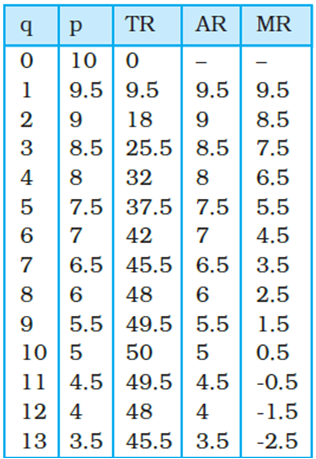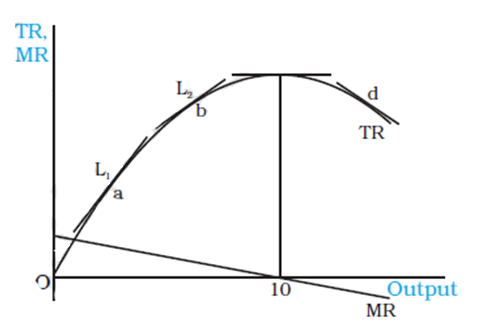Economics (NCERT) Notes

6.3 Total, Average and Marginal Revenues

Price and Revenue of a Monopoly firm•Let the demand function be given by the equation
•q = 20 2p,
•where q is the quantity sold and p is the price in rupees.
Hence, p = 10 0.5q

Total, Average and Marginal Revenues

•TR does not increase by the same amount for every unit increase in quantity.
•As the quantity increases further, the rise in TR is smaller.
•After 10 units of output, TR starts declining.
•Thus bringing more than 10 units for sale leads to a level of TR less than Rs 50.
•This change in Total Revenue (TR) due to the sale of an additional unit represents Marginal Revenue (MR).

Definition of Marginal Revenue
•The change in Total Revenue (TR) due to the sale of an additional unit is termed Marginal Revenue (MR).

Relation between Marginal Revenue and Total Revenue Curves•The marginal revenue at any level of output is given by the slope of the total revenue curve at that level of output.
•At point ‘a’ on the TR curve, the value of MR is given by the slope of the line L1, and at point ‘b’ by the line L2. The line L2 is flatter than line L1, ie its slope is lesser.
•When 10 units of the commodity are sold, the tangent to the TR is horizontal, ie its slope is zero.
•The value of the MR for the same quantity is zero.
•At point ‘d’ on the TR curve, where the tangent is negatively sloped, the MR takes a negative value.
•Hence, when total revenue is rising, marginal revenue is positive, and when total revenue shows a fall, marginal revenue is negative.

Relation between Average Revenue and Marginal Revenue curves

•Fig shows that the MR curve lies below the AR curve
•If the AR curve is steeper, then the MR curve is far below the AR curve.

Marginal Revenue and Price Elasticity of Demand

•The MR values also have a relation with the price elasticity of demand.
•The price elasticity of demand is more than 1 when the MR has a positive value, and becomes less than the unity when MR has a negative value.
•As the quantity of the commodity increases, MR value becomes smaller and the value of the price elasticity of demand also becomes smaller.
•A demand curve is called
•Elastic at a point where price elasticity is greater than unity,
•Inelastic at a point where the price elasticity is less than unity and
•Unitary elastic when price elasticity is equal to 1.
•Table shows that
•when quantity is less than 10 units, MR is positive and the demand curve is elastic and
•when quantity is of more than 10 units, the demand curve is inelastic.
•At the quantity level of 10 units, the demand curve is unitary elastic.

 Related Articles
 • 6.8 Behaviour of Firms in Oligopoly • 6.1 Non-competitive Markets • 5.8 Applications of Supply-Demand Analysis • 5.6 Market Equilibrium: Free Entry and Exit • 5.5 Impact of Shift in Supply and Demand • 5.2 Market Equilibrium for Fixed Number of Firms • 5.1 Market Equilibrium • 4.10 Price Elasticity of Supply • 4.9 Market supply Curve • 8.1 Importance of Infrastructure
 Recent Articles
 • Q9. A journalist fighting the stone mafia • Innovation and Creativity • Love and hatred • Religion and Spirituality • Tulsidas • Bureaucrat at the Temple • Getting Fooled for Kindness • Burning the boat • Three Masons • Two Salesmen